##CS 660: Combinatorial Algorithms Introduction Part A

[To Lecture Notes Index]
San Diego State University -- This page last updated Septemper 3, 1995## References

Introduction To Algorithms, Corman, Leiserson,Rivest, Chapters 1-4

## Mathematical Analysis of Algorithms

### Model of Computing

If analysis of algorithms is the answer, what is the question?

Given two or more algorithms for the same task, which is better?
Under which condition is bubble sort better than insertion sort?

What computing resources does an algorithm require?
How long will it take bubble sort to sort a list of N items?

Goal of mathematical analysis is a function of the resources required of an algorithm

On what computer?
What is a Computer?
Random-access machine (RAM)
Single processor
Instructions executed sequentially
Each operation requires the same amount of time

Single cost vs. Lg(N) cost
Time required for basic operation?
3 + 6
1234!
Insertion Sort
A = - infinity
for K = 2 to N do
begin
J = K;
Key = A[J];
while Key < A[J-1] do
begin
A[J] = A[J-1];
J = J - 1;
end while;
A[J] = Key;
end for;
Complexity
Resources required by the algorithm as a function of the input size

Worst-case Analysis
Complexity of an algorithm based on worst input of each size

Average-case Analysis
Complexity of an algorithm averaged over all inputs of each size
Insertion Sort
```		Comparisons	Element moves

worst case	(N+1)N/2 - 1	(N-1)N/2

average case	(N+1)N/4 - 1/2	(N-1)N/4
```

### Asymptotic Notation

Asymptotically tight bound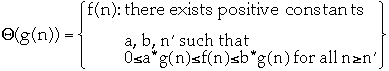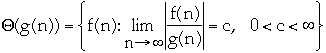Asymptotic upper bounds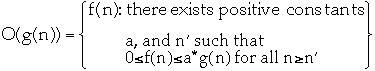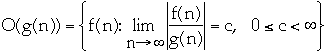Common Myths and Errors
• Everyone incorrectly writes: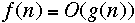instead of: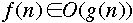•ordoes not mean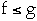or even that there is an n such that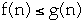Let f(n) = 2n + 10, and g(n) = n then
f(n) = O(g(n)) but f(n) > g(n)

• Using O( ) when mean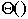Bubble vs. Insertion Sort
```		Worst case	Average case

Bubble sort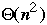Insertion Sort```

Bubble Sort
```		Comparisons	Element moves

worst case	(N-1)N/2	3(N-1)N/2

average case	(N-1)N/2	3(N-1)N/4

best case	(N-1)N/2	0
```

Insertion Sort
```		Comparisons	Element moves

worst case	(N+1)N/2 - 1	(N-1)N/2

average case	(N+1)N/4 - 1/2	(N-1)N/4

best case	N - 1	0
```
Bubble vs. Insertion SortTiming Results
Worst Case
```	N	Bubble	Insertion
100	1	1
200	5	3
400	19	11
800	79	42
1600	317	166
```

Average Case
```	N	Bubble	Insertion
100	1	0
200	3	1
400	14	5
800	56	21
1600	228	84
```

What is wrong with this Picture?